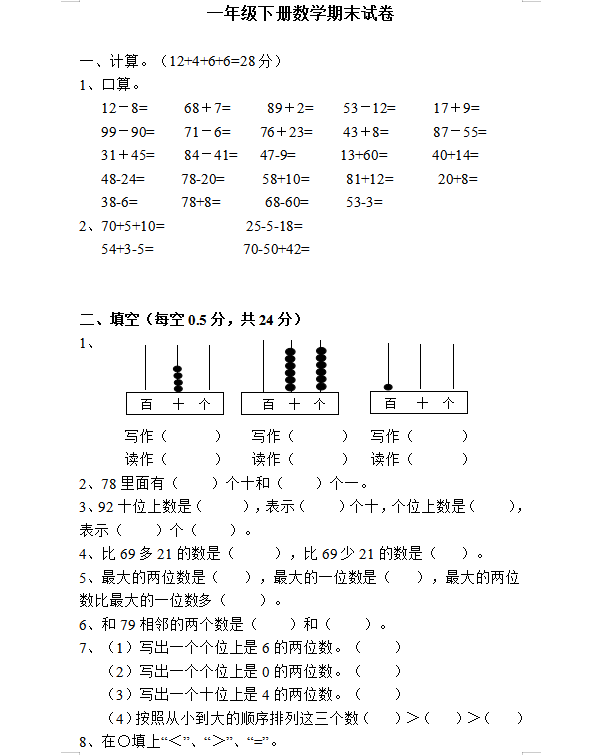1、口算。

12－8=     68＋7=     89＋2=    53－12=     17＋9=

99－90=    71－6=    76＋23=    43＋8=      87－55=

31＋45=    84－41=   47-9=      13+60=      40+14=

48-24=     78-20=     58+10=     81+12=      20+8=